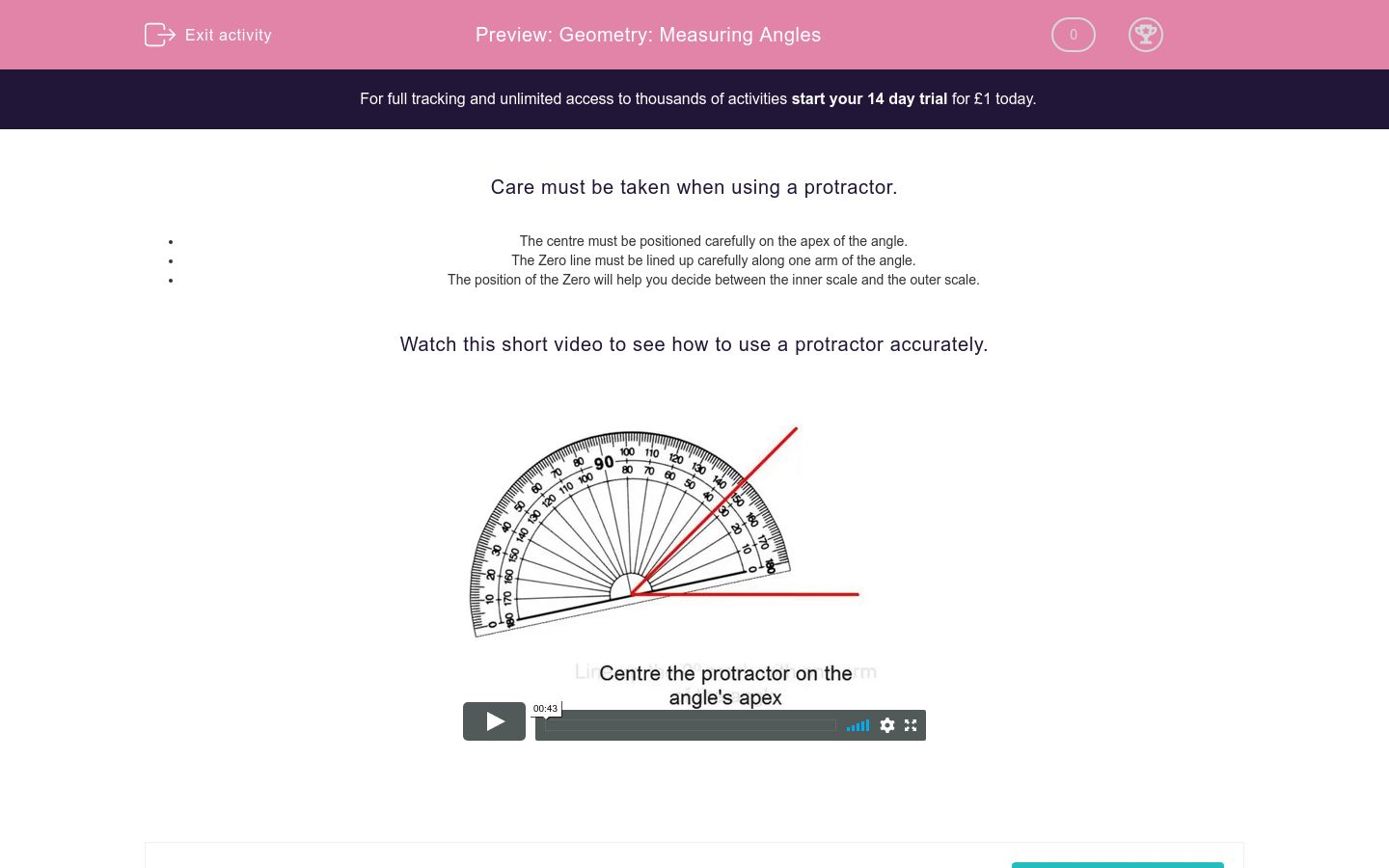# Geometry: Measuring Angles

In this worksheet, students measure angles using the onscreen protractor.Key stage:  KS 2

Curriculum topic:   Maths and Numerical Reasoning

Difficulty level:### QUESTION 1 of 10

Care must be taken when using a protractor.

• The centre must be positioned carefully on the apex of the angle.
• The Zero line must be lined up carefully along one arm of the angle.
• The position of the Zero will help you decide between the inner scale and the outer scale.

Watch this short video to see how to use a protractor accurately.

Use the protractor to measure this angle.

Select the correct angle.70°

110°

180°

Use the protractor to measure this angle.

Select the correct angle.55°

65°

125°

135°

Use the protractor to measure this angle.

Select the correct angle.38°

42°

142°

Use the protractor to measure this angle.

Select the correct angle.80°

100°

180°

Use the protractor to measure this angle.

Select the correct angle.80°

100°

180°

Use the protractor to measure this angle.

Select the correct angle.64°

124°

76°

116°

Use the protractor to measure this angle.

Select the correct angle.65°

75°

115°

125°

Use the protractor to measure this angle.

Select the correct angle.136°

56°

144°

44°

Use the protractor to measure this angle.

Select the correct angle.73°

107°

113°

87°

Use the protractor to measure this angle.

Select the correct angle.48°

152°

32°

148°

• Question 1

Use the protractor to measure this angle.

Select the correct angle.70°
• Question 2

Use the protractor to measure this angle.

Select the correct angle.55°
• Question 3

Use the protractor to measure this angle.

Select the correct angle.38°
• Question 4

Use the protractor to measure this angle.

Select the correct angle.100°
• Question 5

Use the protractor to measure this angle.

Select the correct angle.80°
• Question 6

Use the protractor to measure this angle.

Select the correct angle.116°
• Question 7

Use the protractor to measure this angle.

Select the correct angle.65°
• Question 8

Use the protractor to measure this angle.

Select the correct angle.44°
• Question 9

Use the protractor to measure this angle.

Select the correct angle.107°
• Question 10

Use the protractor to measure this angle.

Select the correct angle.148°
---- OR ----

Sign up for a £1 trial so you can track and measure your child's progress on this activity.

### What is EdPlace?

We're your National Curriculum aligned online education content provider helping each child succeed in English, maths and science from year 1 to GCSE. With an EdPlace account you’ll be able to track and measure progress, helping each child achieve their best. We build confidence and attainment by personalising each child’s learning at a level that suits them.

Get started